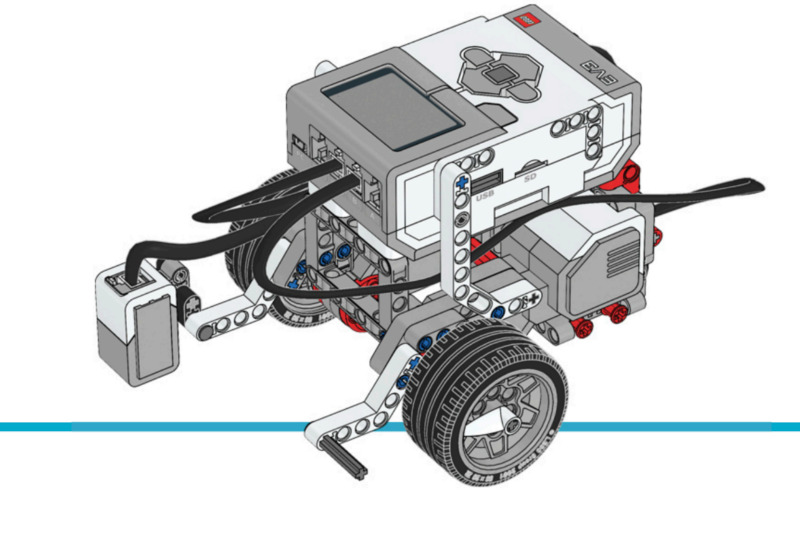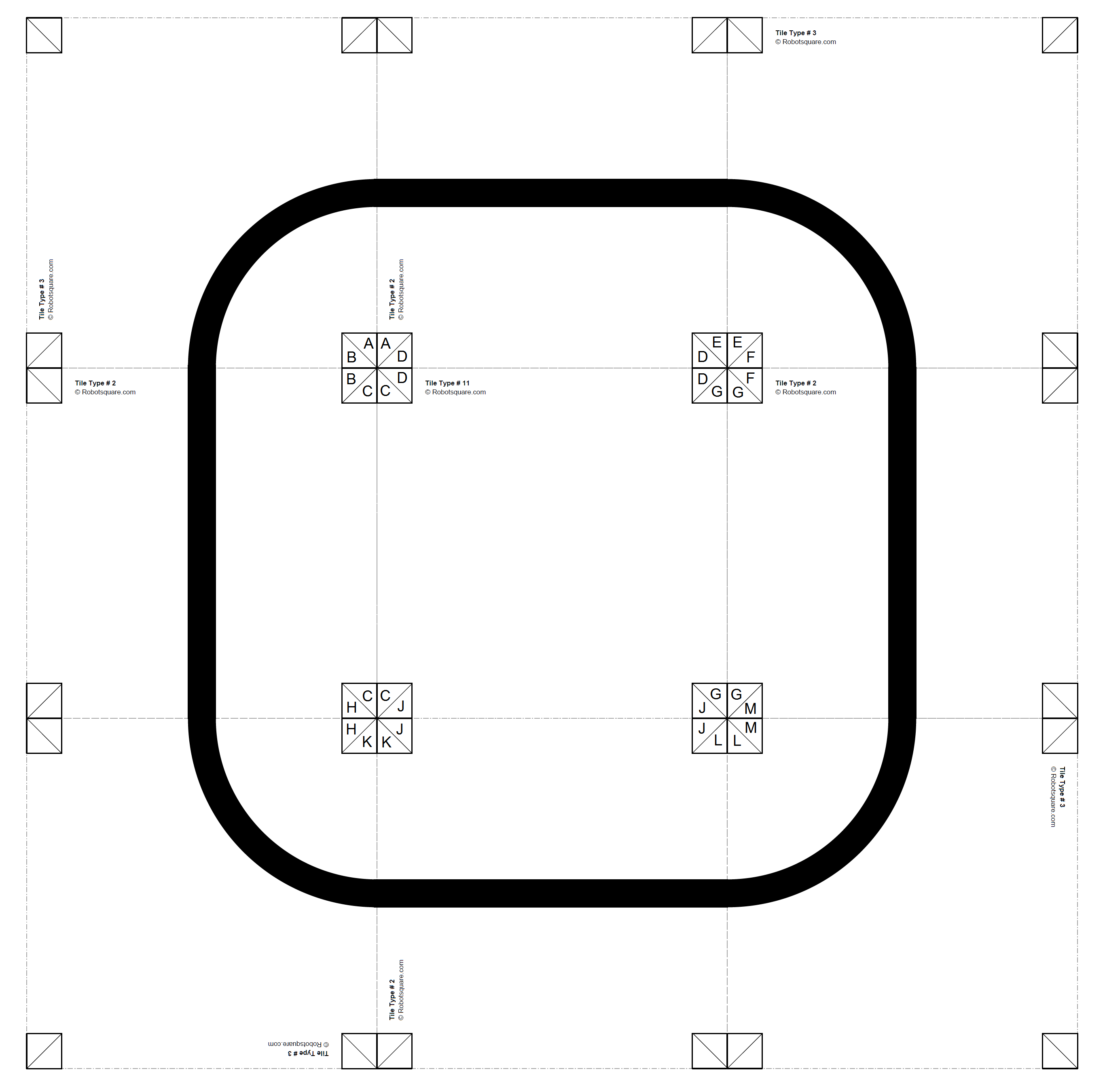# Line Following¶

This example project shows how you can make a robotic vehicle track a line using the ColorSensor and the DriveBase class. This works by adjusting the turn rate based on how much the measured reflection deviates from the threshold value. The threshold value is selected as the average of the line reflection and the reflection of the surrounding surface.

Building instructions

Click here to find all building instructions for the Educator Bot, or use this link to go to the color sensor attachment directly.Figure 25 Robot Educator with the Color Sensor

Example program

This example uses the track shown in Figure 26, but you can adapt this example to follow other lines as well. Download the line following track using the link below and print print the required pages. You can also create your own track by printing other pages.

Click to download the line following track.Figure 26 Download the line following track and print pages 2,2,2,2,3,3,3,3,11.

#!/usr/bin/env pybricks-micropython

"""
Example LEGO® MINDSTORMS® EV3 Robot Educator Color Sensor Down Program
----------------------------------------------------------------------

This program requires LEGO® EV3 MicroPython v2.0.

Building instructions can be found at:
https://education.lego.com/en-us/support/mindstorms-ev3/building-instructions#robot
"""

from pybricks.ev3devices import Motor, ColorSensor
from pybricks.parameters import Port
from pybricks.tools import wait
from pybricks.robotics import DriveBase

# Initialize the motors.
left_motor = Motor(Port.B)
right_motor = Motor(Port.C)

# Initialize the color sensor.
line_sensor = ColorSensor(Port.S3)

# Initialize the drive base.
robot = DriveBase(left_motor, right_motor, wheel_diameter=55.5, axle_track=104)

# Calculate the light threshold. Choose values based on your measurements.
BLACK = 9
WHITE = 85
threshold = (BLACK + WHITE) / 2

# Set the drive speed at 100 millimeters per second.
DRIVE_SPEED = 100

# Set the gain of the proportional line controller. This means that for every
# percentage point of light deviating from the threshold, we set the turn
# rate of the drivebase to 1.2 degrees per second.

# For example, if the light value deviates from the threshold by 10, the robot
# steers at 10*1.2 = 12 degrees per second.
PROPORTIONAL_GAIN = 1.2

# Start following the line endlessly.
while True:
# Calculate the deviation from the threshold.
deviation = line_sensor.reflection() - threshold

# Calculate the turn rate.
turn_rate = PROPORTIONAL_GAIN * deviation

# Set the drive base speed and turn rate.
robot.drive(DRIVE_SPEED, turn_rate)

# You can wait for a short time or do other things in this loop.
wait(10)